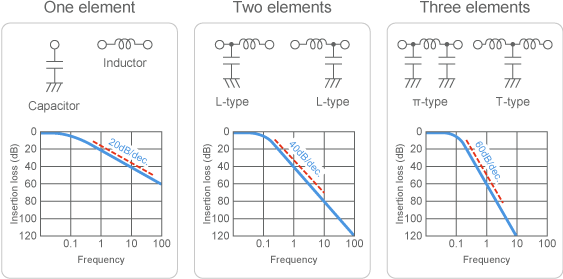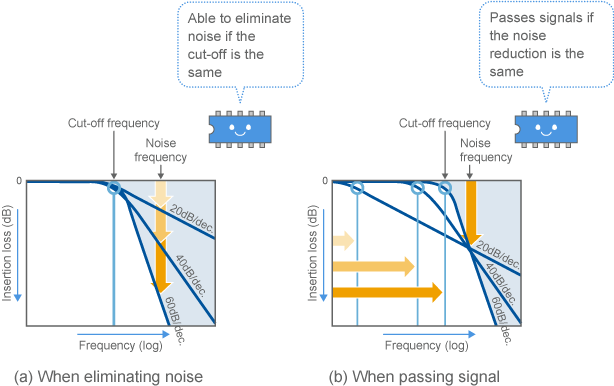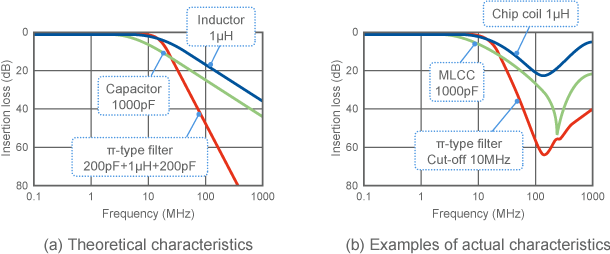EMI Suppression Filters (EMC and Noise Suppression)Noise Suppression Products/EMI Suppression Filters

Noise Suppression Basic Course Section 1
Chapter 1
Chapter 2
Chapter 3
Chapter 4
Chapter 5
Chapter 6
Chapter 6

EMI suppression filters

6-3-6. Filters that combine capacitors and inductors

(1) LC filters

Capacitors and inductors can be combined to improve frequency characteristics. Fig. 8 shows the basic characteristics of some LC filters that combine capacitors and inductors.
If a single capacitor or inductor is used, the frequency characteristics will have a slope of 20dB/dec.
An L-type filter, in which a single capacitor and single inductor are combined, will have a slope of 40dB/dec. A π-type or T-type filter formed from a total of three parts will have a slope of 60dB/dec.

(2) Each part increases the slope 20dB/dec.

The slope of the frequency characteristics can be increased by 20dB by adding another part to the combination. Each part increases the slope 20dB. Doing so can help to improve the filter's ability to pick out signals and noise (Fig. 1).
The number of parts in a filter is referred to as the "order" of the filter. An L-type filter is a second order filter, while a π-type or T-type filter is a third order filter. Filters with higher orders have steeper frequency characteristics.

(3) Alternate capacitors and inductors when combining them

When combining parts, inductors and capacitors are alternated. If two capacitors or inductors are combined together, the order of the filter will not increase. Doing so merely increases the capacitor and inductor constants.
Note that the characteristics shown in Fig. 8 are idealized. If the capacitor and inductor constants are not set appropriately according to the impedance of the surrounding circuit, the frequency characteristics will not slope so steeply.Fig. 8 LC filter configuration and frequency characteristics

(4) Benefits of using LC filters

As shown above, filters with higher orders have steeper frequency characteristic slopes. This property offers the following benefits in suppressing noise. (On the other hand, this is not beneficial in terms of cost as there are more parts involved.)

1. (1) More noise can be eliminated when the cut-off frequency is the same.
2. (2) Signals up to a higher frequency can be passed when the noise reduction capability is the same.
3. (3) An extremely large insertion loss (that would be impossible with a single part) can be obtained.

An explanation of these benefits follows.

・ Easier to separate signals and noise

(1) and (2) are beneficial when the frequency of the signal and frequency of the noise are close. As shown in Fig. 9, this makes it possible to reduce noise while maintaining the signal frequency. LC compound filters are therefore often used for clock signals in which the pulse waveform must be retained.
In order to accurately control the cut-off frequency, the capacitor and inductor constants must be adjusted to match the impedance of the surrounding circuit. Many LC filters prepared for use as filters for signals are adjusted to match a circuit of around 50 ohms.Fig. 9 Benefits of steep frequency characteristics

・Able to greatly attenuate noise

(3) above is beneficial because there is a limit to insertion loss when using a single part.
For example, when eliminating noise with a capacitor, it would be possible to completely eliminate noise (100dB or higher at all frequencies exceeding 1MHz), even if a capacitor with the theoretical maximum electrostatic capacitance was used (1000μF, for example). In reality however, a single capacitor is only capable of obtaining an insertion loss of around 60dB at some frequencies, no matter how large the electrostatic capacitance of the capacitor is (not counting three-terminal capacitors and other special capacitors explained later). This is because capacitors possess parasitic elements such as ESR and ESL, in addition to electrostatic capacitance.
This limit can be overcome by combining a capacitor with an inductor. An insertion loss of, for example, 80dB (or even over 100dB depending on the conditions) is possible with an LC filter.
This is why LC filters are used in switching power supplies and other very noisy devices.

6-3-7. Examples of actual filter characteristics

(1) Comparison of filters with a cut-off around 10MHz

What are the real characteristics of capacitors and inductors? Fig. 10 shows an example comparing the characteristics of three types of filters with cut-off frequencies around 10MHz. Shown here are a capacitor, inductor, and π-type LC filter.

(2) Theoretical characteristics

Fig. 10 (a) shows the theoretical values introduced above. To make the graphs easier to read, the capacitor and inductor constants are truncated. Closely matching the constants (for example, setting the inductor to 2.5μH) would cause the curves of the capacitor and inductor to completely overlap. The cut-off frequency of the π-type filter is around 16MHz.

(3) Actual part characteristics

Fig. 10 (b) demonstrates an example of actual part characteristics. Nominal values are given for each part, so some measurement errors are included. Also, the π-type filter constant is different from the value used in the calculation in (a). Even so, the figure shows that values very close to the theoretical characteristics can actually be obtained for frequencies at and below 100MHz.
On the other hand, actual characteristics diverge significantly from theoretical values for frequencies exceeding 100MHz. The insertion loss value in particular drops suddenly around 1GHz.
This is because the effect of parasitic elements on capacitors and inductors is stronger in higher frequencies. The effect of parasitic elements will be covered in the next section.Fig. 10 Theoretical and actual characteristics

6-3-8. Summary of low-pass filters using LC

1. (1) Capacitors and inductors are used as elements for low-pass filters.
2. (2) Capacitors bypass noise currents to ground.
3. (3) Inductors choke noise currents.
4. (4) Combining capacitors and inductors can improve frequency characteristics.

Low-pass filters using LC" - Key points

• Capacitors and inductors are used as elements for low-pass filters.
• Capacitors bypass noise currents to ground.
• Inductors choke noise currents.
• Combining capacitors and inductors can improve frequency characteristics.